# KSEEB SSLC Class 10 Maths Solutions Chapter 9 Polynomials Ex 9.3

In this chapter, we provide KSEEB SSLC Class 10 Maths Solutions Solutions Chapter 9 Polynomials Ex 9.3 for English medium students, Which will very helpful for every student in their exams. Students can download the latest KSEEB SSLC Class 10 Maths Solutions Solutions Chapter 9 Polynomials Ex 9.3 pdf, free KSEEB SSLC Class 10 Maths Solutions Solutions Chapter 9 Polynomials Ex 9.3 pdf download. Now you will get step by step solution to each question.

### Karnataka State Syllabus Class 10 Maths SolutionsChapter 9 Polynomials Ex 9.3

Question 1.
Divide the polynomial p(x) by the polynomial g(x) and find the quotient and remainder in each of the : following.
(i) p(x) = x3 – 3x2 + 5x – 3; g(x) = x2 – 2
(ii) p(x) = x4 – 3x2 + 4x + 5; g(x) = x2 + 1 – x
(iii) p(x) = x4 – 5x + 6; g(x) = 2 – x2.
Solution:
(i) p(x) = x3 – 3x2 + 5x – 3
g(x) =x2 – 2
q(x) = ?
r(x) = ?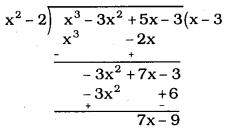∴ Quotient, q(x) = (x – 3)
Remainder, r(x) = (7x – 9)

(ii) p(x) = x4 – 3x2 + 4x + 5
g(x) = x2 + 1 – x
q(x) = ?
r(x) = ?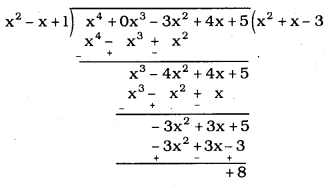∴ Quotient, q(x) = x2 + x – 3
Remainder, r(x) = 8

(iii) p(x) = x4 – 5x + 6
g(x) = 2 – x2
q(x) = ?
r(x) = ?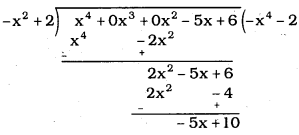∴ Quotient, q(x) = -x2 – 2
Remainder, r(x) = -5x + 10

Question 2.
Check whether the first polynomial is a factor of the second polynomial by dividing the second polynomial by the first polynomial :
(i) t2 – 3; 2t4 + 3t3 – 2t2 – 9t – 12
(ii) x2 + 3x + 1; 3x4 + 5x3 – 7x2 + 2x + 2
(iii) x3 – 3x + 1; x5 – 4x3 + x2 + 3x + 1
Solution:
(i) t2 – 3; 2t4 + 3t3 – 2t2 – 9t – 12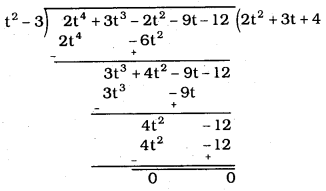∴ t2 – 3 is the factor of 2t4 + 3t3 – 2t2 – 9t – 12 because remainder is zeroes

(ii) x2 + 3x + 1; 3x4 + 5x3 – 7x2 + 2x + 2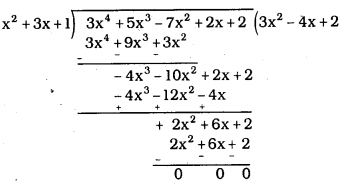Here remainder is zero
∴ x2 + 3x + 1 is the factor of 3x4 + 5x3 – 7x2 + 2x + 2

(iii) x3 – 3x + 1; x5 – 4x3 + x2 + 3x + 1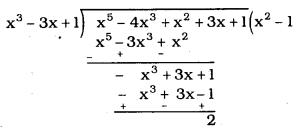Here remainder r(x) ≠ 0 ∵ 2 ≠ 0
∴ x3 – 3x + 1 is not the factor of x5 – 4x3 + x2 + 3x + 1

Question 3.
Obtain all other zeroes of 3x4 + 6x3 – 2x2 – 10x – 5, if two of its zeroes are 53−−√ and −53−−√
Solution:
Roots of expressions are 53−−√ and −53−−√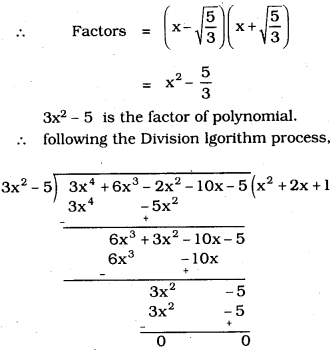∴ In q(x) = x2 + 2x + 1, there are two more roots.
x2 + 2x + 1 = (x + 1)2
other roots are: x = -1, -1

Question 4.
On dividing x3 – 3x2 + x + 2 by a polynomial g(x), the quotient and remainder were x – 2 and -2x + 4, respectively. Find g(x).
Solution:
x3 – 3x2 + x + 2 = g(x) × (x – 2) + (-2x + 4)
g(x) × (x – 2) = x3 – 3x2 + x + 2 – (-2x + 4)
= x3 – 3x2 + x + 2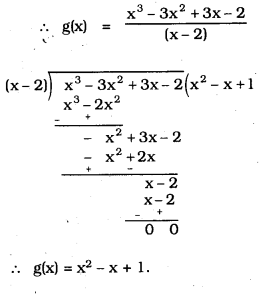Question 5.
Give examples of polynomials p(x), g(x), q(x) and r(x), which satisfy the division algorithm and
(i) deg p(x) = deg q(x)
(ii) deg q(x) = deg r(x)
(iii) deg r(x) = 0
Solution:
i) P(x) = 2x2 – 2x + 14, g(x) = 2,
q(x) = x2 – x + 7 and r(x) = 0
Verification
P(x) = g(x) q(x) + r(x)
2x2 – 2x + 14 = 2(x2 – x + 7) + 0
= 2x2 – 2x + 14
∴ LHS = RHS

ii) P(x) = x3 + x2 + x + 1, g(x) = x2 – 1, q(x) = x + 1 and r(x) = 2x + 2
Verification
P(x) = q(x) g(x) + r(x)
x3 + x2 + x + 1 =(x+ 1) (x2 – 1) + 2x + 2
= x3 – x + x2 – 1 + 2x + 2
= x3 + x2 + x + 1
LHS = RHS

iii) P(x) = x3 + 2x2 – x + 2, g(x) = x2 – 1, q(x) = x + 2 and r(x) = 4
p(x) = g(x) q(x) + r(x)
x3 + 2x2 – x + 2 = (x2 – 1) (x + 2) + 4
= x3 + 2x2 – x – 2 + 4
= x3 + 2x2 – x + 2
LHS = RHS

All Chapter KSEEB Solutions For Class 10 Maths

—————————————————————————–

All Subject KSEEB Solutions For Class 10

*************************************************

I think you got complete solutions for this chapter. If You have any queries regarding this chapter, please comment on the below section our subject teacher will answer you. We tried our best to give complete solutions so you got good marks in your exam.

If these solutions have helped you, you can also share kseebsolutionsfor.com to your friends.

Best of Luck!!